© | Dror Bar-Natan: Classes: 2009-10: AKT:

# Video 090910-1

 width: 320 720 download ogg/AKT-090910-1_320.ogg For now, this video can only be viewed with web browsers that support

Videography by Karene Chu

Notes on AKT-090910-1:    [edit, refresh]

3-colourings, Reidemeister's theorem, invariance, the Kauffman bracket.

# Week of... Videos, Notes, and Links
: 3-colourings, Reidemeister's theorem, invariance, the Kauffman bracket.
: R23 invariance of the bracket, R1, the writhe, the Jones polynomial, programming the Jones polynomial.
Tricolourability
2 Sep 14 : More on Jones, some pathologies and more on Reidemeister, our overall agenda.
: The definition of finite type, weight systems, Jones is a finite type series.
: The skein relation for Jones; HOMFLY-PT and Conway; the weight system of Jones.
3 Sep 21 : FI, 4T, HOMFLY and FI and 4T, statement of the Fundamental Theorem, framed knots.
: Some dimensions of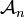${\mathcal A}_n$,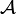${\mathcal A}$ is a commutative algebra,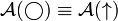${\mathcal A}(\bigcirc)\equiv{\mathcal A}(\uparrow)$.
Class Photo
:${\mathcal A}$ is a co-commutative algebra, the relation with products of invariants,${\mathcal A}$ is a bi-algebra.
4 Sep 28 Homework Assignment 1
Homework Assignment 1 Solutions
: The Milnor-Moore theorem, primitives, the map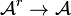${\mathcal A}^r\to{\mathcal A}$.
: Jacobi diagrams, AS, IHX, STU, and the equivalence of all that with 4T.
: The very basics on Lie algebras.
5 Oct 5 : Lie algebraic weight systems,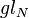$gl_N$.
: More on$gl_N$, Lie algebras and the four colour theorem.
: The "abstract tenssor" approach to weight systems,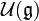${\mathcal U}({\mathfrak g})$ and PBW, the map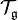${\mathcal T}_{\mathfrak g}$.
6 Oct 12 : Algebraic properties of${\mathcal U}({\mathfrak g})$ vs. algebraic properties of${\mathcal A}$.
Thursday's class canceled.
7 Oct 19 : Universal finite type invariants, filtered and graded spaces, expansions.
Homework Assignment 2
The Stonehenge Story
: The Stonehenge Story to IHX and STU.
: The Stonhenge Story: anomalies, framings, relation with physics.
8 Oct 26 : Knotted trivalent graphs and their chord diagrams.
: Zsuzsi Dancso on the Kontsevich Integral (1).
: Zsuzsi Dancso on the Kontsevich Integral (2).
9 Nov 2 : The details of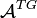${\mathcal A}^{TG}$.
: Three basic problems: genus, unknotting numbers, ribbon knots.
: The three basic problems and algebraic knot theory.
10 Nov 9 : Tangles and planar algebras, shielding and the generators of KTG.
Homework Assignment 3
No Thursday class.
11 Nov 16 Local Khovanov Homology
: Local Khovanov homology, I.
: Local Khovanov homology, II.
12 Nov 23 : Emulation of one structure inside another, deriving the pentagon.
: Peter Lee on braided monoidal categories, I.
: Peter Lee on braided monoidal categories, II.
13 Nov 30 : The relations in KTG.
: The Existence of the Exponential Function.
: The Final Exam, Dror's failures.
F Dec 7 The Final Exam on Thu Dec 10, 9-11, Bahen 6183.
Register of Good Deeds / To Do List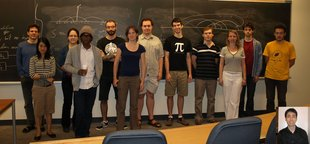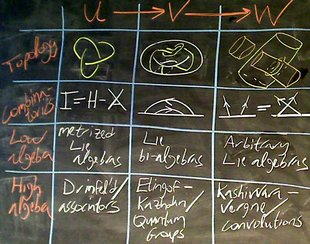refresh
panel
Managed by dbnvp: Click "Comment on h:mm:ss" below the video to add a comment on a specific time.0:02:53  Mathematics begins:0:03:05  1st math item: Want to prove Trefoil knot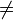$\ne$ Unknot (via Tricolorability).0:03:59  The handout "Some Non-Obvious Examples" (of the difficulty of distinguishing knots) is here. The purpose was to convince us that what we are trying to prove (Trefoil knot$\ne$ Unknot) is not as obvious as it might seem.0:06:35  Knot tabulation: The knot table of all prime knots with up to 10 crossings used to have 251 entries but now has 250, since in the 1970's Perko proved that two of them are the same (see ).0:08:18  Define invariant of knots: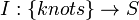$I: \left\{knots\right\}\rightarrow S$, where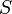$S$ is a set. Our approach to prove Trefoil knot$\ne$ Unknot: to construct an invariant that distinguishes these two knots.0:10:26  Construction/definition of such an invariant (simplest for our purpose), tricolourability, on knot diagrams.0:10:29  Knot diagram: a 'nice' projection of a knot onto a plane (at most double points are allowed and all intersections are transverse).0:11:48  As a start, a function on knot diagrams rather than on knots is defined: For a knot diagram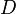$D$,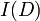$I(D)$ gives the truth value of the following statement:

The arcs of$D$ can be coloured in 3 colours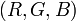$(R,G,B)$ s.t.

1. Every crossing is either monochromatic or trichromatic.
2. All colours appear.0:16:25  Proving: the (particular planar projection of the) trefoil knot is tricolourable whereas (the planar diagram of) the unknot is not.0:18:44  Want to prove: tricolourability is a knot invariant. Quoting and explaining the Reidemeister Theorem, which is relied upon in the proof.0:23:54  Reducing the problem: via the Reidemeister Theorem, to proving that tricolourability is invariant under each of the 3 Reidemeister moves.0:24:20  The Reidemeister Theorem tells us that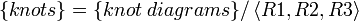$\{knots\}=\{knot \; diagrams\}/\left\langle R1,R2,R3 \right\rangle$.0:26:06  Sketch of proof that tricolourability is invariant under all 3 Reidemeister moves:0:26:16  Invariance of tricolourability under R1. There are 2 directions to check, i.e. (left) 3-colourable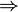$\Rightarrow$ (right) 3-colourable, and (left) colourable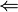$\Leftarrow$ (right) colourable. Side benefit: explanation of 'local' diagram notation.0:29:24  Invariance of tricolourability under R2. For each of the 2 directions (as in the proof for R1), there are cases (corresponding to different colourings of, say the top, 'external endpoints' of the local diagram modulo colour permutations; the colouring of all arcs will then be forced by the local rule of tricolourability and the fact that we are only dealing with three colours, and the cases which violate the global rule should be discarded) to check.

Terminology (for the purpose of this annotation)
Local rule: at each crossing, of the 3 arcs involved, either 1 or all 3 colours appear.
Global rule: all 3 colours must appear.
(Together with the provision of 3 colours, knots which can be coloured obeying these rules, are called tricolourable as defined at 0:10:26 of this hour.)0:33:25  Cont'd: Invariance of tricolourability under R2. In particular, the subtlety (as viewed locally) about the total number of colours used (globally) is discussed.

Problem/Concern: Sometimes, in a local picture, only 2 colours appear on one side of an isotopy move (e.g. R2) whereas all 3 colours appear on the other. One might worry that this could lead to the violation of the global rule.

Solution: One can prove that a knot (consisting of only 1 connected piece of material in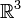$\mathbb{R}^3$), which is coloured obeying the local rule of tricolourability, has at least 2 colours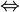$\Leftrightarrow$ it has all 3 colours. The proof relies on the fact that the same piece of material can change colour (from one colour to a 2nd colour) only by going 'under' a crossing, and whenever a crossing involves 2 colours it must involve a 3rd. (This argument fails, however, for links. Just consider two knots, one red and one blue say, placed side by side.)0:36:07  Invariance of tricolourability under R3 can be proved similarly (as for R2). There are no losses of colours locally (in some sense simpler). However, there are many more colour combinations, well just 5 up to permutation of colours, to check (perhaps this is the reason why Dror thinks the proof is boring).0:36:19  End of 1st math item: Q.E.D. Trefoil knot$\ne$ Unknot.0:37:00  2nd math item: Towards introducing a much stronger knot invariant, the Jones polynomial.0:37:17  Obvious limitation of Tricolourability: It only takes two possible values, so definitely cannot distinguish between three knots. Hence, we desire a stronger invariant which can get us closer to the goal of distinguishing the many different knots listed in the Rolfsen table.0:39:37  Historical remarks about the Jones polynomial (discovered in the 80s) and the related Kauffman bracket (later found to simplify the description of the Jones polynomial).0:40:07  Defining the Kauffman bracket or simply the bracket as a polynomial in$A$,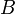$B$, and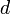$d$. It is recursively defined on links.0:41:23  I messed up and switched between 0- and 1-smoothings! Here's the correct version, taken from my paper Khovanov's Homology for Tangles and Cobordisms:0:45:11  The Kauffman bracket is completely determined by the relations:

1. Failed to parse (unknown function\slashoverback): \left\langle\slashoverback\right\rangle=A\left\langle\hsmoothing\right\rangle + B \left\langle\smoothing\right\rangle
1.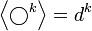$\left\langle \bigcirc^k \right\rangle = d^k$

These suffice because for any knot or link diagram we can apply the first relation recursively until we eliminate all the crossings and end up with a disjoint union of unknots. For instance, we get:

Failed to parse (unknown function\HopfLink): \left\langle \HopfLink \right\rangle = A^2d^2 + 2ABd + B^2d^20:46:24  Cont'd: Defining the last axiom (about unlinks) of the Kauffman bracket.0:47:35  Example: Computing the Kauffman bracket of the Hopf link.0:51:06  Preview of the 2nd hour: Will derive relations between$A$,$B$ and$d$, in order for the bracket polynomial to be invariant under the Reidemeister moves.